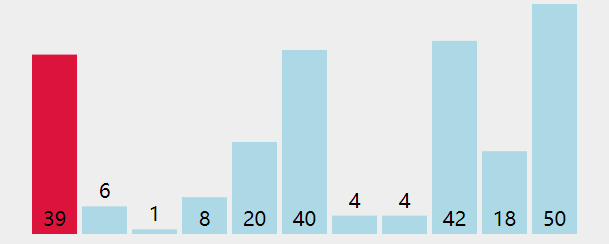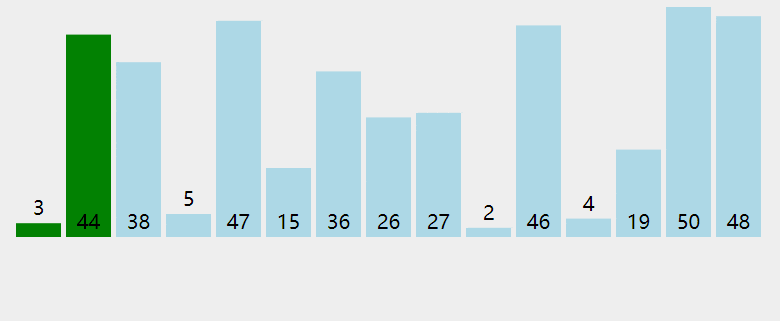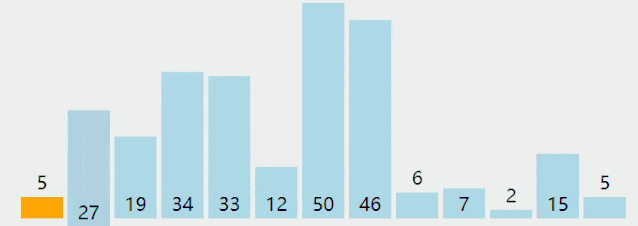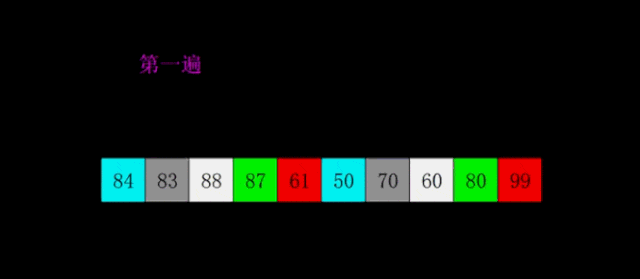# 常见的初级排序算法，这次全搞懂Silently9527## 排序算法的模板

public interface SortTemplate {    void sort(Comparable[] array);    default void print(Comparable[] array) {        for (Comparable a : array) {            System.out.print(a + " ");        }    }    default boolean less(Comparable a, Comparable b) {        return a.compareTo(b) < 0;    }    default void exch(Comparable[] array, int i, int j) {        Comparable tmp = array[i];        array[i] = array[j];        array[j] = tmp;    }    }

• Comparable: 为了让我们实现的排序算法更加的通用，可以排序任意的对象，所以我们这里使用了 Comparable 数组

• sort: 不同的排序算法实现的方式不一样，子类自己去实现

• less: 定义的公用方法，如果 a < b 就返回 true

• exch: 定义的公用方法，交换数组中的两个对象

• print: 打印出数据中的每个元素

## 选择排序

• 首先找到数组中的最小元素，

• 其实将它和数组中的第一个元素进行交换，这样就排定了一个元素；

• 再次找出剩余元素中最小的元素与数组中的第二个元素进行交换，如此反复直到所有元素都是有序的public class SelectionSort implements SortTemplate {    @Override    public void sort(Comparable[] array) {        int length = array.length;        for (int i = 0; i < length; i++) {            int min = i;            for (int j = i + 1; j < length; j++) {                if (less(array[j], array[min])) {                    min = j;                }            }            exch(array, i, min);        }    }}

## 冒泡排序

• 比较相邻的两个元素，如果前一个比后一个大，那么就交换两个元素的位置

• 对每一组相邻的元素执行同样的操作，直到最后一个元素，操作完成之后就可以排定一个最大的元素

• 如此往复，直到数组中所有的元素都有序public class BubbleSort implements SortTemplate {    @Override    public void sort(Comparable[] array) {        int length = array.length - 1;        for (int i = 0; i < length; i++) {            for (int j = 0; j < length - i; j++) {                if (less(array[j + 1], array[j])) {                    exch(array, j, j + 1);                }            }        }    }}

## 插入排序

• 初始默认第一个元素就是有序的，当前索引的位置从 0 开始

• 先后移动当前索引的位置，当前索引位置左边的元素是有序的，从后往前开始扫码与当前索引位置元素进行比较

• 当确定当前索引位置上的元素在左边有序适合的位置之后，插入到该位置上

• 如果当确定当前索引位置上的元素大于了已排序的最后一个元素，那么当前索引位置直接往后移动

• 如此反复，直到所有元素有序public class InsertionSort implements SortTemplate {    @Override    public void sort(Comparable[] array) {        int length = array.length;        for (int i = 1; i < length; i++) {            for (int j = i; j > 0 && less(array[j], array[j - 1]); j--) {                exch(array, j, j - 1);            }        }    }}

## 希尔排序

• 首先设置一个步长 h 用来分隔出子数组

• 用插入排序将 h 个子数组独立排序

• 减小 h 步长继续排序子数组，直到 h 步长为 1

• 当步长为 1 时就成了普通的插入排序，这样数组一定是有序的public class ShellSort implements SortTemplate {    @Override    public void sort(Comparable[] array) {        int gap = 1;        int length = array.length;        while (gap < length / 3) {            gap = 3 * gap + 1;        }        while (gap >= 1) {            for (int i = gap; i < length; i++) {                for (int j = i; j >= gap && less(array[j], array[j - gap]); j -= gap) {                    exch(array, j, j - gap);                }            }            gap = gap / 3;        }    }}

## 最后（点关注，不迷路）### Silently9527

Simple Programmer, Make the complex simple

## 评论